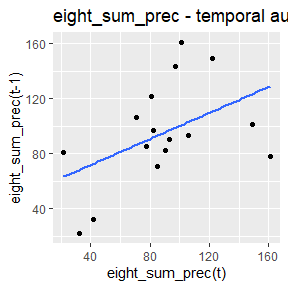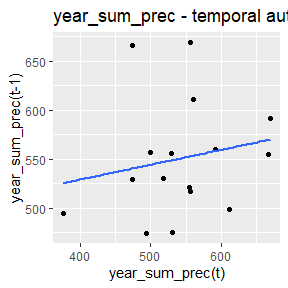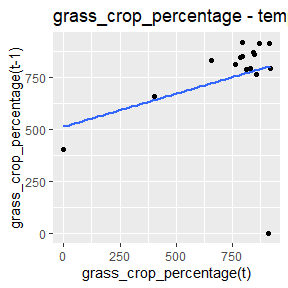# dynamicSDM: Model fitting and autocorrelation

library(dynamicSDM)

## Stage 3: Explanatory variable data

In this tutorial, we will be fitting boosted regression tree SDMs to our dataset, whilst accounting for spatial and temporal autocorrelation.

## Import covariates

The covariate data frame generated in the second tutorial can be imported from your project directory.

project_directory <- file.path(file.path(tempdir(), "dynamicSDM_vignette"))
# project_directory<-"your_path_here"
dir.create(project_directory)
#> Warning in dir.create(project_directory):
#> exists

#sample_explan_data <- read.csv(paste0(project_directory, "/extracted_quelea_occ.csv"))

Or alternatively, you can run the code below to read the pre-extracted data into your R environment from the dynamicSDM package.

data("sample_explan_data")

### a) Test for spatial and temporal autocorrelation

Autocorrelation is when explanatory variable data for species records taken closer in space and time are more similar to each other than to those of records more distantly sampled. When species distribution modelling with spatio-temporally dynamic explanatory variables, spatial and temporal autocorrelation can impact model performance.

Run the code below to test for spatial and temporal autocorrelation in the extracted explanatory variable data using spatiotemp_autocorr(). This function can also generate a temporal autocorrelation plot for each variable.

variablenames<-c("eight_sum_prec","year_sum_prec","grass_crop_percentage")

autocorrelation <- spatiotemp_autocorr(sample_explan_data,
varname = variablenames,
plot = TRUE,
temporal.level = c("year")) # can choose month or day too
#> geom_smooth() using formula = 'y ~ x'#> geom_smooth() using formula = 'y ~ x'#> geom_smooth() using formula = 'y ~ x'

autocorrelation
#> $Statistical_tests #>$Statistical_tests$eight_sum_prec #>$Statistical_tests$eight_sum_prec$Temporal_autocorrelation
#> $Statistical_tests$eight_sum_prec$Temporal_autocorrelation$year
#>
#>  Pearson's product-moment correlation
#>
#> data:  first_obs and second_obs
#> t = 1.9975, df = 14, p-value = 0.06558
#> alternative hypothesis: true correlation is not equal to 0
#> 95 percent confidence interval:
#>  -0.03229165  0.78370005
#> sample estimates:
#>       cor
#> 0.4709523
#>
#>
#>
#> $Statistical_tests$eight_sum_prec$Spatial_autocorrelation #> observed expected sd p.value #> 1 -0.01417411 -0.003039514 0.001844916 1.586784e-09 #> #> #>$Statistical_tests$year_sum_prec #>$Statistical_tests$year_sum_prec$Temporal_autocorrelation
#> $Statistical_tests$year_sum_prec$Temporal_autocorrelation$year
#>
#>  Pearson's product-moment correlation
#>
#> data:  first_obs and second_obs
#> t = 0.71217, df = 14, p-value = 0.4881
#> alternative hypothesis: true correlation is not equal to 0
#> 95 percent confidence interval:
#>  -0.3402656  0.6247751
#> sample estimates:
#>       cor
#> 0.1869775
#>
#>
#>
#> $Statistical_tests$year_sum_prec$Spatial_autocorrelation #> observed expected sd p.value #> 1 -0.09107117 -0.003039514 0.001845888 0 #> #> #>$Statistical_tests$grass_crop_percentage #>$Statistical_tests$grass_crop_percentage$Temporal_autocorrelation
#> $Statistical_tests$grass_crop_percentage$Temporal_autocorrelation$year
#>
#>  Pearson's product-moment correlation
#>
#> data:  first_obs and second_obs
#> t = 1.2466, df = 14, p-value = 0.233
#> alternative hypothesis: true correlation is not equal to 0
#> 95 percent confidence interval:
#>  -0.2129836  0.7018300
#> sample estimates:
#>      cor
#> 0.316094
#>
#>
#>
#> $Statistical_tests$grass_crop_percentage$Spatial_autocorrelation #> observed expected sd p.value #> 1 -0.1581672 -0.003039514 0.001848495 0 #> #> #> #>$Plots
#> $Plots$eight_sum_prec
#> $Plots$eight_sum_prec$year #> geom_smooth() using formula = 'y ~ x'#> #> #>$Plots$year_sum_prec #>$Plots$year_sum_prec$year
#> geom_smooth() using formula = 'y ~ x'#>
#>
#> $Plots$grass_crop_percentage
#> $Plots$grass_crop_percentage$year #> geom_smooth() using formula = 'y ~ x'### b) Accounting for spatial and temporal autocorrelation One approach to account for autocorrelation when species distribution modelling is to split records into sampling units based upon spatial and temporal factors, and then group units into separate blocks so that the mean and standard deviation of ecoclimatic variables are roughly equal across blocks. SDMs are then fitted in a jack-knife approach, leaving out each block in-turn to use as the test dataset. spatiotemp_block() splits occurrence records into sampling units by spatial categories (e.g. ecoregions or biome) and temporal units (e.g. month or year of record), before blocking the data. As some spatial categories can be very large, the argument spatial.split.degrees can be used to split large contiguous regions into smaller units. In our case study, we use a raster of various biomes in southern Africa and split large biomes by 3 degree cells. The function returns the original data frame with an additional column containing the block numbers that each record belongs to. data("sample_biome_data") sample_explan_data <- spatiotemp_block(sample_explan_data, spatial.layer = sample_biome_data, spatial.split.degrees = 3, vars.to.block.by = variablenames, temporal.block = "month", n.blocks = 3, iterations = 5000) ### c) Fit species distribution models There are many SDM approaches to modelling the relationships between species occurrence and associated explanatory variables. dynamicSDM includes the function brt_fit() to fit boosted regression tree models to training and test data. There are arguments to specify the blocks to split data by (block.col, function returns a list of models the length of unique blocks) and to weight records by spatio-temporal sampling effort (weights.col, see the Stage 1 tutorial). sample_explan_data$weights <- (1 - sample_explan_data\$REL_SAMP_EFFORT)

models <- brt_fit(sample_explan_data,
response.col = "presence.absence",
varnames = variablenames,
block.col = "BLOCK.CATS",
weights.col = "weights",
distribution = "bernoulli",
interaction.depth = 2)
##### Note:

If you want to use other modelling approaches, this data frame containing response and explanatory variable data can easily be input into SDM functions in other packages.

## Summary

At the end of this vignette, we now have our fitted species distribution models for each spatio-temporal block. Let’s save this list of models to our project directory for use in the next tutorial!

saveRDS(models, file = paste0(project_directory, "/fitted_quelea_SDMs.rds"))